Sie sind hier: ICP » R. Hilfer » Publikationen

# 4 Approximate Analytical Solution

## 4.1 Step Function Approximation

[2330.2.1] The basic idea of the analysis below is to approximate the travelling wave profile for long times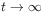with piecewise constant functions (step functions). [2330.2.2] For large [page 2331, §0]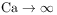(i.e. in the Buckley-Leverett limit) one may view this approximate profile as a superposition of two Buckley-Leverett shock fronts. [2331.0.1] This is possible by virtue of the fact, that the Heaviside step function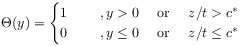(35)

can also be regarded as a function of the similarity variable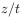of the Buckley-Leverett problem.

[2331.1.1] In the crudest approximation one can split the total profile for sufficiently large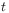into the sum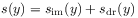(36a)

of an imbibition front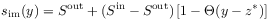(36b)

located at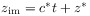and a drainage front profile located at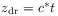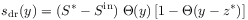(36c)

both moving with the same speed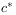, where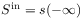resp.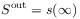are the upper (inlet) resp. lower (outlet) saturations and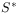is the maximum (overshoot) saturation. [2331.1.2] The quantity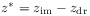is the distance by which the imbibition front precedes the drainage front, i.e. the width of the tip (=overshoot) region.

## 4.2 Travelling wave solutions

[2331.2.1] The two equations (28) become coupled, if eq.  (20) holds true, because then there is only a single wave speedfor both fronts. [2331.2.2] At the imbibition discontinuity the Rankine-Hugoniot condition demands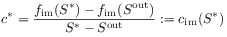(37a)

and the second equality (with colon) defines the function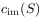. [2331.2.3] Similarly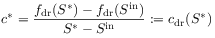(37b)

defines the drainage front velocity as a function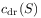of the overshoot. Examples of the velocities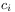used in the compuations are shown in Figure 1a. [2331.2.4] For a travelling wave both fronts move with the same velocity so that the mathematical problem is to find a solutionof the equation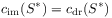(38)

obtained from equating eqs. (37b) and (37a) (See Fig. 1a). [2331.2.5] The wave velocityis then obtained as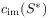or equivalently as.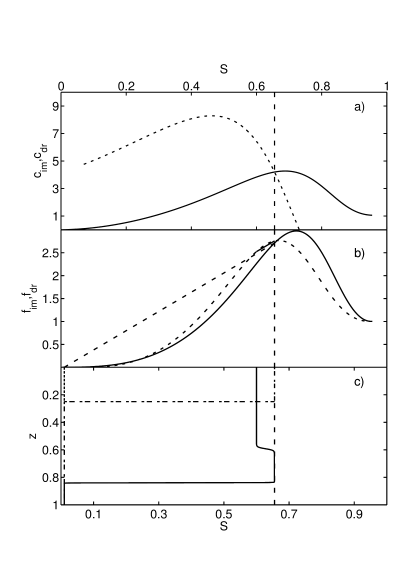Figure 1: a) Graphical solution of eq.  (38). The dashed curve shows, the solid line shows. b) Fractional flow functions for drainage (dashed) and imbibition (solid). The two secants (solid/dashed) show the graphical construction of the travelling wave solution with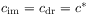. For better visibility, their line styles have been reversed. Their slopes are equal to each other. c) Numerical solution of eq. (29) using Open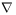FOAM for the travelling wave constructed graphically in subfigure b). The solution is displayed at time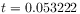. The monotone initial profile at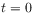is shown as a dash-dotted step.

[page 2332, §1]

## 4.3 General overshoot solutions with two wave speeds

[2332.1.1] Equation (38) provides a necessary condition for the existence of a travelling wave solution of the form of eq.  (36) with velocityand overshoot. [2332.1.2] More generally, if the saturation plateau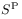is larger or smaller than, one expects to find non-monotone profiles that are, however, not travelling waves. [2332.1.3] Instead the drainage and imbibition fronts are expected to have different velocities. [2332.1.4] The fractional flow functions with relative permeabilities from eqs. (31) and the parameters from Table 1 give for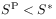the result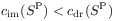(39)

while for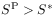one has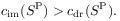(40)

[2332.1.5] In this case, for plateau saturations, the leading (imbibition) front has a smaller velocity than the trailing (drainage) front. [2332.1.6] Thus the trailing front catches up and the profile approaches a single front at long times. [2332.1.7] For plateau saturationson the other hand the trailing drainage front moves slower than the leading imbibition front. [2332.1.8] In this case a non-monotone profile persists indefinitely, albeit with a plateau (tip) width that increases linearly with time.

Parameter Symbol Value Units
system size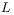1.0 m
porosity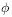0.38
permeability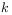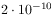m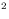density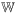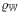1000 kg/m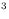density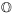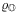800 kg/mviscosity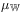0.001 Pa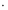s
viscosity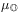0.0003 Pas
imbibition exp.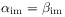0.85
drainage exp.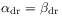0.98
end pnt. rel.p.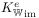0.35
end pnt. rel.p.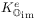1
end pnt. rel.p.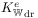0.35
end pnt. rel.p.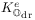0.75
imb. cap. press.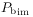55.55 Pa
dr. cap. press.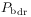100 Pa
end pnt. sat.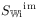0
end pnt. sat.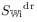0.07
end pnt. sat.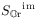0.045
end pnt. sat.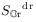0.045
boundary sat.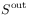0.01
boundary sat.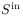0.60
total flux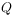1.196 10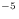m/s
Table 1: Parameter values, their symbols and units